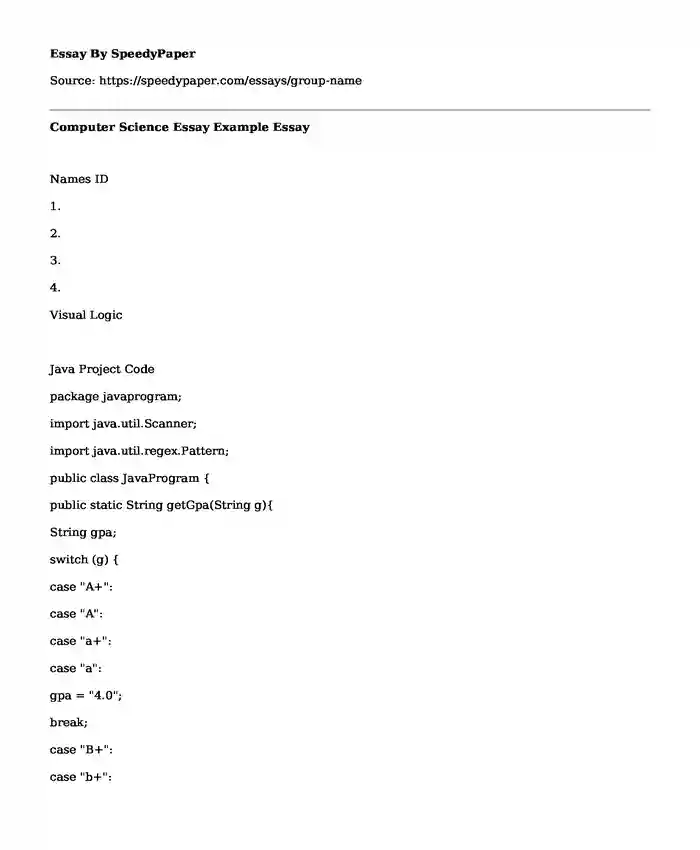# Computer Science Essay Example

Published: 2019-09-11Categories: Computer science Software Pages: 3 Wordcount: 560 words
143 views

Names IDIs your time best spent reading someone else’s essay? Get a 100% original essay FROM A CERTIFIED WRITER!

1.

2.

3.

4.

Visual Logic

Java Project Code

package javaprogram;

import java.util.Scanner;

import java.util.regex.Pattern;

public class JavaProgram {

public static String getGpa(String g){

String gpa;

switch (g) {

case "A+":

case "A":

case "a+":

case "a":

gpa = "4.0";

break;

case "B+":

case "b+":

gpa = "3.75";

break;

case "B":

case "b":

gpa = "3.5";

break;

case "C+":

case "c+":

gpa = "3.0";

break;

case "C":

case "c":

gpa = "2.5";

break;

case "D+":

case "d+":

gpa = "2.0";

break;

case "D":

case "d":

gpa = "1.0";

break;

case "F":

case "f":

gpa = "0.0";

break;

default:

break;

}

return gpa;

}

public static void main(String[] args) {

String gpa;

System.out.println();

System.out.print("please enter credit hours for Course Code1-"+code1+":");

System.out.println();

System.out.print("please enter credit hours for Course Code2-"+code2+":");

System.out.println();

System.out.print("please enter credit hours for Course Code3-"+code3+":");

//Check to ensure valid input of hours

String pattern = "[+-]?[\\d,]+\\.?\\d+";

if(Pattern.matches(pattern, credit1) == true){

System.out.println();

System.out.println("You entered INVALID credit hour for course code1, please check and retry again.");

return;

}

if(Pattern.matches(pattern, credit2) == true){

System.out.println();

System.out.println("You entered INVALID credit hour for course code2, please check and retry again.");

return;

}

if(Pattern.matches(pattern, credit3) == true){

System.out.println();

System.out.println("You entered INVALID credit hour for course code3, please check and retry again.");

return;

}

//Get Course codes, Credit hours and grade

String [] courseCodes = {code1,code2,code3};

String [] courseCredit = {credit1,credit2,credit3};

//Calculate total GPA points

//float [] gpaArr = {gpa1,gpa2,gpa3};

float gpa1TotalPoints = gpa1 * (Float.parseFloat(credit1));

float gpa2TotalPoints = gpa2 * (Float.parseFloat(credit2));

float gpa3TotalPoints = gpa3 * (Float.parseFloat(credit3));

float [] gpaArr = {gpa1,gpa2,gpa3};

//Calculate CGPA

float cgpa1 = gpa1TotalPoints/(Float.parseFloat(credit1));

float cgpa2 = gpa2TotalPoints/(Float.parseFloat(credit2));

float cgpa3 = gpa3TotalPoints/(Float.parseFloat(credit3));

float [] cgpaArr = {cgpa1,cgpa2,cgpa3};

System.out.println("Course code \t\t\t Credit Hours \t\t\t Grade \t\t\t CGPA");

for(int x = 0; x<gpaArr.length; ++x){

System.out.println(courseCodes[x]+" \t\t\t\t "+courseCredit[x]+" \t\t\t\t "+courseGrade[x]+" \t\t\t "+cgpaArr[x]);

}

}

}

Program OutPut